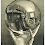## Tuesday, March 17, 2009

### Next in Line

What's the next number?

483, 759, 264, 837, ?

1.Here is the pattern I could find:

Think of this as a long string of digits, ignoring the commas:
483759264837???

Instead of separating this string into groups of three (as above), separate it into groups of four:
4837, 5926, 4837, ???...

There is a pretty clear pattern here: the four-digit number 4837 is followed by the four-digit number 5926, then the sequence is repeated. So the completed sequence would be:
4837, 5926, 4837, 592...

Which, in three-digit groupings, is:
483, 759, 264, 837, 592

So the next number is 592.

Note:
The sequence 48375926... can be broken into two digit groups as well, with a more visible pattern, and yielding the same result:
48,
37 (subtract 11)
26 (subtract 33),
37 (subtract 11),
26 (subtract 33),
and so forth.

2.You found the pattern! I thought this one would stump everyone. I was stumped the first time I saw it, so I thought it was very difficult.

3.This comment has been removed by the author.

4.483, 759, 264, 837

first numbers last 2 digits (83) and seconds numbers first digit (7) make fourth (837), so fifth will be second numbers last two digits (59) and third numbers first digit (2) so 592,

for same reason third numbers last digit (4) is first numbers first digit. number before first will end on 26. and the first digit of number before first will be 9. seconds numbers last digit. number before first one is 926

Leave your answer or, if you want to post a question of your own, send me an e-mail. Look in the about section to find my e-mail address. If it's new, I'll post it soon.

Please don't leave spam or 'Awesome blog, come visit mine' messages. I'll delete them soon after.

Enter your Email and join hundreds of others who get their Question of the Day sent right to their mailbox

The Lamplight Manor Puzz 3-D